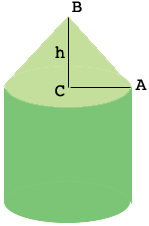SEARCH HOMEMath Central Quandaries & QueriesQuestion from Berania: A water tower in New York City has the shape of a cylinder with a cone on top. The cylinder has a diameter of 12 feet and a height of 15 feet. The roof has an inclination angle of $25^{o}.$ (a) Determine the height of the cone to the nearest tenth of a foot. (b) Determine the overall volume of the tower to the nearest cubic foot.Hi Berania,

The key to this problem is the height of the cone. In my diagram I have labeled the height as $h.$The distance from $C$ to $A$ is 6 fet and the measure of the angle $CAB$ is $25^{o}.$ The triangle $ABC$ is a right triangle.

What trig function relates the lengths of the sides $BC$ and $CA$ to the measure of the angle $CAB?$

PennyMath Central is supported by the University of Regina and The Pacific Institute for the Mathematical Sciences.